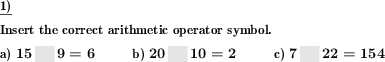Custom math worksheets at your fingertips# Details for problem "Insert the arithmetic operator"

Quickname: 4680

Elementary School, Primary School, Junior High School, Middle School, High School.

## Summary

In an equation with two operands, insert the correct arithmetic symbol.

## Example## Description

An equation with two operands is given. It can be one of these types of statements:

• Subtraction operation
• Multiplication operation
• Division operation

The correct arithmetic operator symbol has to be chosen and put in the blank space so that the equation is correct.

The number of problems and the range of the operators can be chosen.

For the types of operation that can appear,

• add, subtract, multiply and divide

can be chosen.

Download free printable worksheets for this math problem here. The worksheet contains the problems only, the solution sheet includes the answers. Just click on the respective link.

•Worksheet 1Solution sheet with answers
•Worksheet 2Solution sheet with answers
•Worksheet 3Solution sheet with answers

If you can not see the solution sheets for download, they may be filtered out by an ad blocker that you may have installed. If this is the case, please allow ads for this page and reload the page. The solution sheets will then reappear.

• Do these sample worksheets do not really fit?
• Do you need more math worksheets, with a different level of difficulty?
• Would you like to combine different problems on a worksheet and adjust them to your needs?
• As a teacher, you can put together your own worksheets using the automatically generated math problems provided.
With a free initial credit, you can start creating your own math worksheets in a few minutes.

You can try it for free! Register here, to create custom worksheets now!

## Customization options for this problem

Parameter
Possible values
Number of problems
1, 2, 3, 4, 5, 6, 7, 8, 9, 10
Operands number range
10, 20, 30, 40, 50, 100, 200, 500, 1000, 10000, 100000
Operators

## Similar problems

Remark
Description
Insert plus or minus symbol, possible symbols given
In a series of addition or subtraction problems, the plus or minus symbol is missing.
With two operators and three operands
In an equation with three operands, insert two operators.